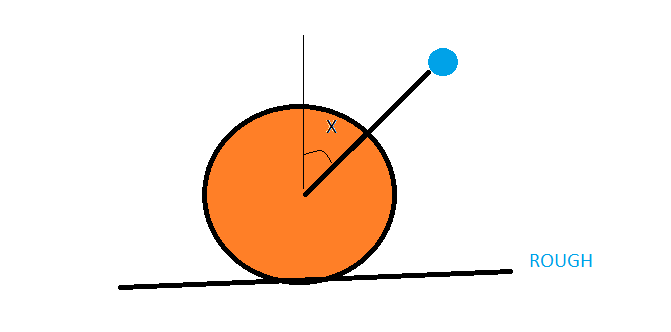# Friction in collisionA large sphere rests on a rough horizontal ground,

A ball of mass 'm' is thrown towards it along one of its radius at a speed 'u' , along an angle 'x' with the vertical

Find the angle with the vertical at which the ball should strike (find x) so that the large sphere pure rolls immediately after collision.

if the answer can be expressed as arctan(x) then find x.

Collision need not be necessarily elastic

Details:
coefficient of friction=1
mass of large sphere = 1
radius of large sphere = sqroot(0.4)

Note:
1)consider the coefficient of friction to be constant during the instant of collision which is not true but assume for this question
2)Treat ground as immovable infinitely heavy object as usual

×

Problem Loading...

Note Loading...

Set Loading...